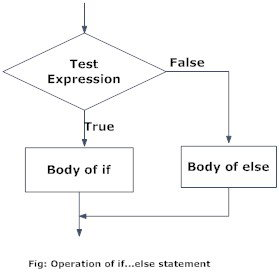# if...else Statement

### What is if...else statement in Python?

Decision making is required when we want to execute a code only if a certain condition is satisfied.
The `if…elif…else` statement is used in Python for decision making.

#### Python if Statement Syntax

if test expression:
statement(s)
Here, the program evaluates the `test expression` and will execute statement(s) only if the test expression is `True`.
If the test expression is `False`, the statement(s) is not executed.
In Python, the body of the `if` statement is indicated by the indentation. The body starts with an indentation and the first unindented line marks the end.
Python interprets non-zero values as `True`. `None` and `0` are interpreted as `False`.

#### Python if Statement Flowchart

Flowchart of if statement in Python programmingFlowchart of if statement in Python programming

#### Example: Python if Statement

# If the number is positive, we print an appropriate message
num = 3
if num > 0:
print(num, "is a positive number.")
print("This is always printed.")
num = -1
if num > 0:
print(num, "is a positive number.")
print("This is also always printed.")
When you run the program, the output will be:
3 is a positive number
This is always printed
This is also always printed.
In the above example, `num > 0` is the test expression.
The body of `if` is executed only if this evaluates to `True`.
When the variable num is equal to 3, test expression is true and statements inside the body of `if` are executed.
If the variable num is equal to -1, test expression is false and statements inside the body of `if` are skipped.
The `print()` statement falls outside of the `if` block (unindented). Hence, it is executed regardless of the test expression.

### Python if...else Statement

#### Syntax of if...else

if test expression:
Body of if
else:
Body of else
The `if..else` statement evaluates `test expression` and will execute the body of `if` only when the test condition is `True`.
If the condition is `False`, the body of `else` is executed. Indentation is used to separate the blocks.

#### Python if..else Flowchart

Flowchart of if...else statement in PythonFlowchart of if...else statement in Python Programming

#### Example of if...else

# Program checks if the number is positive or negative
# And displays an appropriate message
num = 3
# Try these two variations as well.
# num = -5
# num = 0
if num >= 0:
print("Positive or Zero")
else:
print("Negative number")
Output
Positive or Zero
In the above example, when num is equal to 3, the test expression is true and, the body of `if` is executed and the `body` of else is skipped.
If num is equal to -5, the test expression is false, and the body of `else` is executed and the body of `if` is skipped.
If num is equal to 0, the test expression is true and, the body of `if` is executed and `body` of else is skipped.

### Python if...elif...else Statement

#### Syntax of if...elif...else

if test expression:
Body of if
elif test expression:
Body of elif
else:
Body of else
The `elif` is short for else if. It allows us to check for multiple expressions.
If the condition for `if` is `False`, it checks the condition of the next `elif` block and so on.
If all the conditions are `False`, the body of else is executed.
Only one block among the several `if...elif...else` blocks is executed according to the condition.
The `if` block can have only one `else` block. But it can have multiple `elif` blocks.

#### Flowchart of if...elif...else

Flowchart of if...elif....else statement in PythonFlowchart of if...elif....else in Python programming

#### Example of if...elif...else

'''In this program,
we check if the number is positive or
negative or zero and
display an appropriate message'''
num = 3.4
# Try these two variations as well:
# num = 0
# num = -4.5
if num > 0:
print("Positive number")
elif num == 0:
print("Zero")
else:
print("Negative number")
When variable num is positive, Positive number is printed.
If num is equal to 0, Zero is printed.
If num is negative, Negative number is printed.

### Python Nested if statements

We can have a `if...elif...else` statement inside another `if...elif...else` statement. This is called nesting in computer programming.
Any number of these statements can be nested inside one another. Indentation is the only way to figure out the level of nesting. They can get confusing, so they must be avoided unless necessary.

#### Python Nested if Example

'''In this program, we input a number
check if the number is positive or
negative or zero and display
an appropriate message
This time we use nested if statement'''
num = float(input("Enter a number: "))
if num >= 0:
if num == 0:
print("Zero")
else:
print("Positive number")
else:
print("Negative number")
Output 1
Enter a number: 5
Positive number
Output 2
Enter a number: -1
Negative number
Output 3
Enter a number: 0
Zero